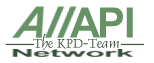Allapi Network API-Guide ApiViewer API Resources Tips & Tricks VB Tutorials Error Lookup Misc Stuff VB examples VB Tools VB Links Top Downloads This Site Search Engine Contact Form

The GradientFill function fills rectangle and triangle structures.

 VB4-32,5,6Declare Function GradientFillTriangle Lib "msimg32" Alias "GradientFill" (ByVal hDC As Long, pVertex As TRIVERTEX, ByVal dwNumVertex As Long, pMesh As GRADIENT_TRIANGLE, ByVal dwNumMesh As Long, ByVal dwMode As Long) As Long

 Operating Systems Supported
 Requires Windows 2000 or later; Requires Windows 98 or later

 Library
 Msimg32

 Parameter Information
 · hdc[in] Handle to the destination device context.· pVertex[in] Pointer to an array of TRIVERTEX structures that each define a triangle vertex.· dwNumVertex[in] The number of vertices in pVertex.· pMesh[in] Array of GRADIENT_TRIANGLE structures in triangle mode, or an array of GRADIENT_RECT structures in rectangle mode.· dwNumMesh[in] The number of elements (triangles or rectangles) in pMesh.· dwMode[in] Specifies gradient fill mode. This parameter can be one of the following values.GRADIENT_FILL_RECT_H In this mode, two endpoints describe a rectangle. The rectangle is defined to have a constant color (specified by the TRIVERTEX structure) for the left and right edges. GDI interpolates the color from the top to bottom edge and fills the interior.GRADIENT_FILL_RECT_V In this mode, two endpoints describe a rectangle. The rectangle is defined to have a constant color (specified by the TRIVERTEX structure) for the top and bottom edges. GDI interpolates the color from the top to bottom edge and fills the interior.GRADIENT_FILL_TRIANGLE In this mode, an array of TRIVERTEX structures is passed to GDI along with a list of array indexes that describe separate triangles. GDI performs linear interpolation between triangle vertices and fills the interior. Drawing is done directly in 24- and 32-bpp modes. Dithering is performed in 16-, 8-, 4-, and 1-bpp mode.

 Return Values
 If the function succeeds, the return value is TRUE.If the function fails, the return value is FALSE.

 Examples

 Related Functions